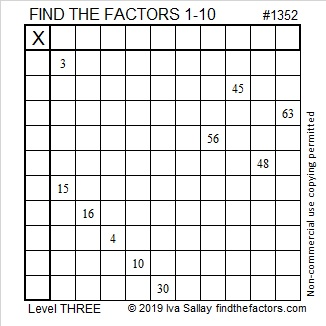# 1352 and Level 3

If you know what numbers divide evenly into both 3 and 15, then you can solve this puzzle. Only use factor pairs where both numbers are from 1 to 10. Start at the top of the puzzle and work down cell by cell until you have found all the factors!Print the puzzles or type the solution in this excel file: 10 Factors 1347-1356

Here I explain how to solve the above puzzle:

Here are a few facts about the puzzle number, 1352:

• 1352 is a composite number.
• Prime factorization: 1352 = 2 × 2 × 2 × 11 × 11, which can be written 1352 = 2³ × 13²
• 1352 has at least one exponent greater than 1 in its prime factorization so √1352 can be simplified. Taking the factor pair from the factor pair table below with the largest square number factor, we get √1352 = (√676)(√2) = 26√2
• The exponents in the prime factorization are 3 and 2. Adding one to each exponent and multiplying we get (3 + 1)(2 + 1) = 4 × 3 = 12. Therefore 1352 has exactly 12 factors.
• The factors of 1352 are outlined with their factor pair partners in the graphic below.1352 is the hypotenuse of two Pythagorean triples:
520-1248-1352 which is (5-12-13) times 104
952-960-1352 which is 8 times (119-120-169)